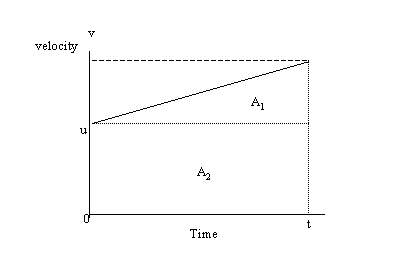# Solutions to SQA examination

### Paper I Solutions

```1. D		11. D			21. D
2. B		12. C			22. A
3. C		13. C			23. B
4. C		14. B			24. E
5. C		15. D			25. B
6. A		16. C			26. C
7. D		17. A			27. A
8. D		18. B			28. D
9. D		19. A			29. D
10.E		20. A			30. E

31.The displacement(s) is equal to the total area(A1+A1) under
the graph.

s = A1+A1
s = ut +(v-u)t/2

Note that from the definition of acceleration :

a = (v-u)/t
=>(v-u)= at

Substitute this into the expression above.

s = ut + att/2
s = ut + at2/2 as required.

32.	Density of  air = Mass of air released/Volume of air released

r = Mair/Vair

Mair = (363.86-362.00)g = 1.86g = 1.86x10-3kg
Vair = 1687.00cm3 = 1687.00x10-6m3

r = 1.86x10-3kg/1687.00x10-6m3
r = 1.1025kg/m3

33.a.	T(K) = T(oC) + 273
T(K) = -196 + 273
T(K) = 77K

b.	The kelvin temperature is directly proportional to the kinetic
energy of particles. At 0K particles have no kinetic energy. A lower
energy state/temperature, is therefore, not possible.

34.a.	Vinverting input = 0V

b.	I = V/R
V = 0.5V
R = 10,000W

I = 0.5/10000
I = 50mA

35.	l = v/f
l = 340/1000
l = 0.34m

Path difference = (1.37-1.20)m = 0.17m

This means that loudspeaker 2 is a distance of 0.17m further away
from the microphone than loudspeaker 1. This is a distance equivalent
to l/2. Therefore, the waves reach the microphone 180o out of phase
and interfere destructively, reducing the amplitude of the sound.

36.a.	All metals and carbon are conductors.
Plastic and rubber are examples of insulators.
Group IV elements such as silicon are semiconductors.

b.	By adding pentavalent (group V) atoms to group IV semiconductor, n-type
semiconductor material is made. This material has a lower resistance than
pure semiconductor, as a result of an excess of negative charge carriers
in the structure.

37.a.	When two atoms join, or fuse together, to produce a single atom of greater mass
the reaction is called fusion.

b. 	The mass of the product of a fusion reaction is less than that of the
initial reactants. This "missing mass" is converted into energy.

E = mc2

Where:	m = missing mass
c = speed of light

END OF QUESTION PAPER